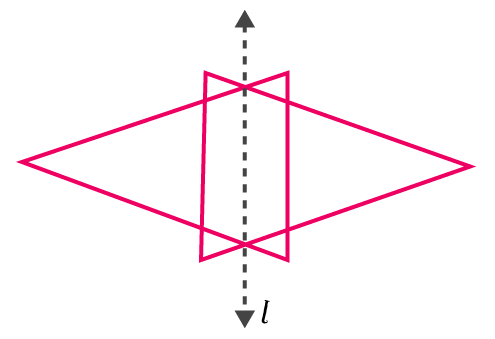## Access NCERT Solutions for Class 6 Chapter 13: Symmetry Exercise 13.1

### Access NCERT Solutions for Class 6 Chapter 13: Symmetry Exercise 13.1

1. List any four symmetrical objects from your home or school.

Solutions:

The four symmetrical objects are black board, the table top, a pair of scissors, the computer disc etc.

2. For the given figure, which one is the mirror line, l1 or l2?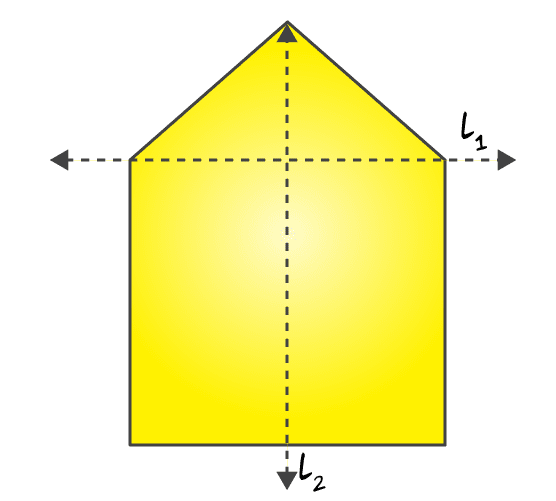Solutions:

l2 is the mirror line of the figure. When given figure is folded about the line l2 the left part exactly cover the right part and vice versa

3. Identify the shapes given below. Check whether they are symmetric or not. Draw the line of symmetry as well.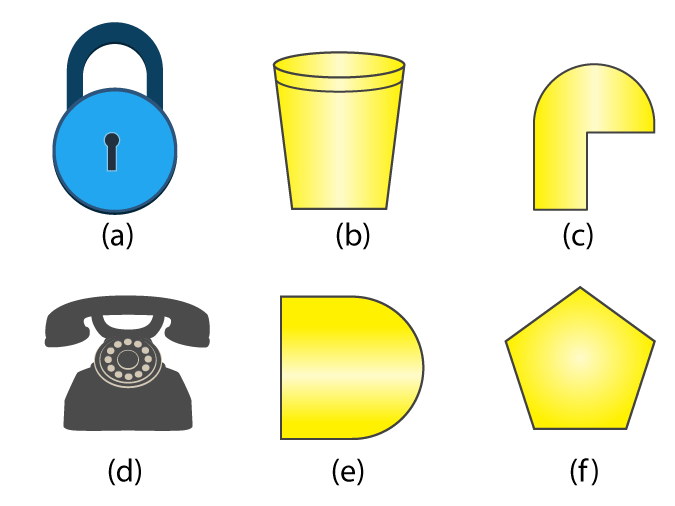Solutions:

(a) Yes. It is a symmetric

(b) Yes. It is a symmetric

(c) No, it is not a symmetric

(d) Yes. It is a symmetric

(e) Yes. It is a symmetric

(f) Yes. It is a symmetric

The following figures given below are the line of symmetry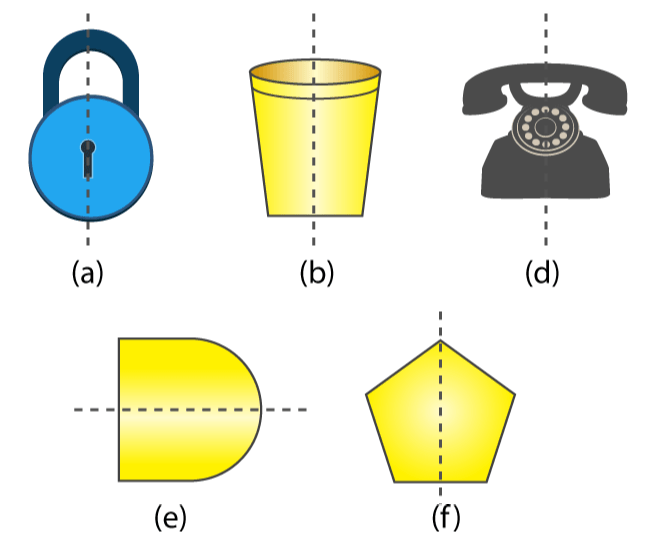4. Copy the following on a squared paper. A square paper is what you would have used in your arithmetic notebook in earlier classes. Then complete them such that the dotted line is the line of symmetry.Solutions:

To make the dotted line as a line of symmetry the given figure may be drawn as follows

(a)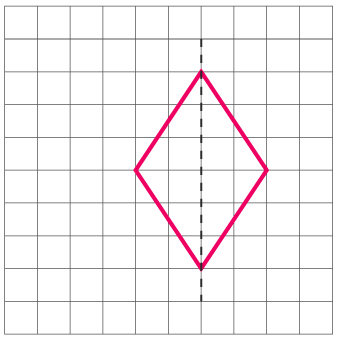(b)(c)(d)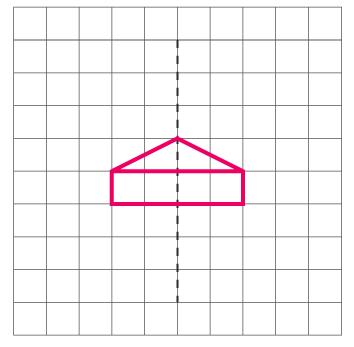(e)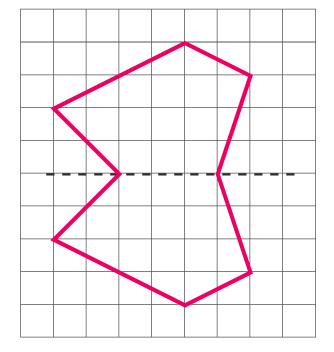(f)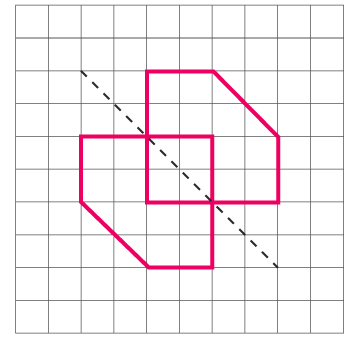5. In the figure, l is the line of symmetry. Complete the diagram to make it symmetric.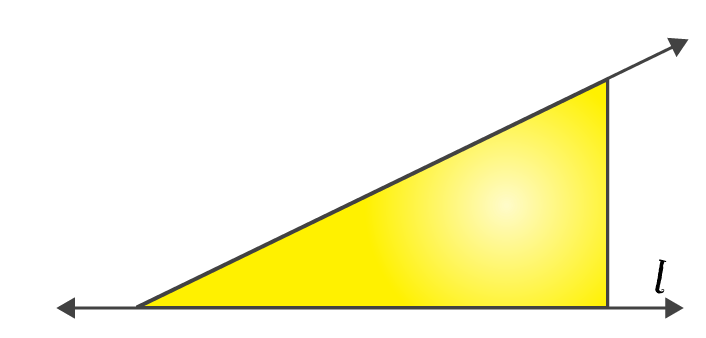Solutions:

The below figure is the complete diagram to make it symmetric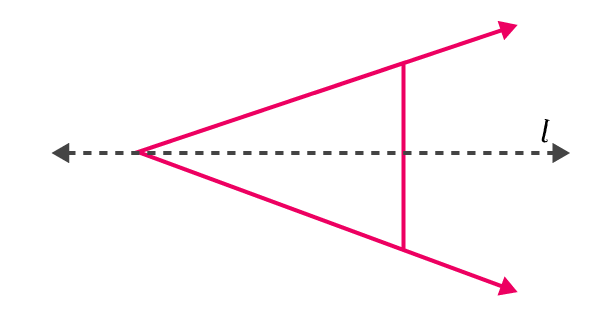6. In the figure, l is the line of symmetry. Draw the image of the triangle and complete the diagram so that it becomes symmetric.Solutions:

The required triangle may be drawn as follow to make it symmetric